# SHAPE SPACE Pythagorean Theorem Pythagorean Theorem Pythagorean Theorem

• Slides: 12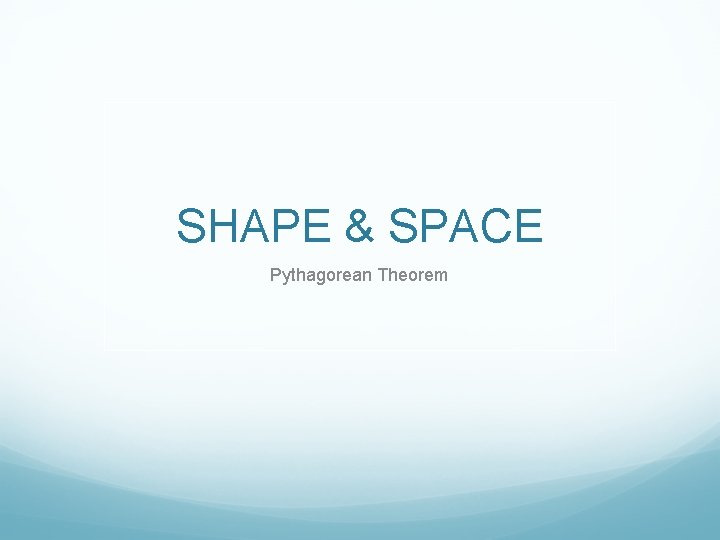SHAPE & SPACE Pythagorean Theorem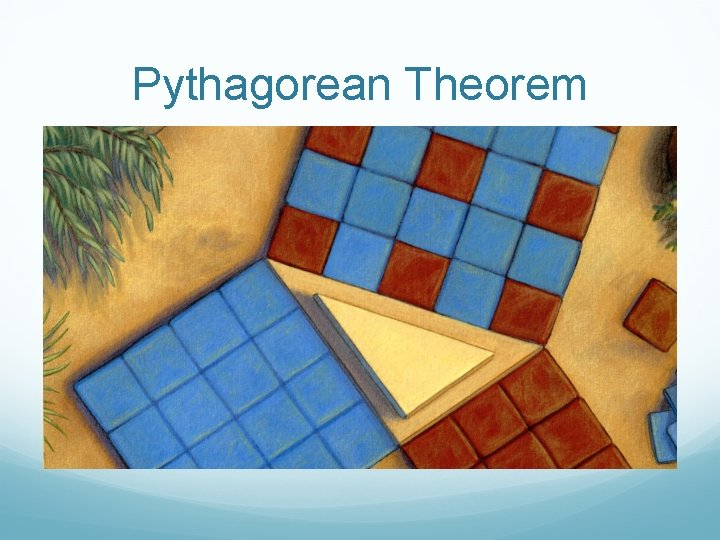Pythagorean Theorem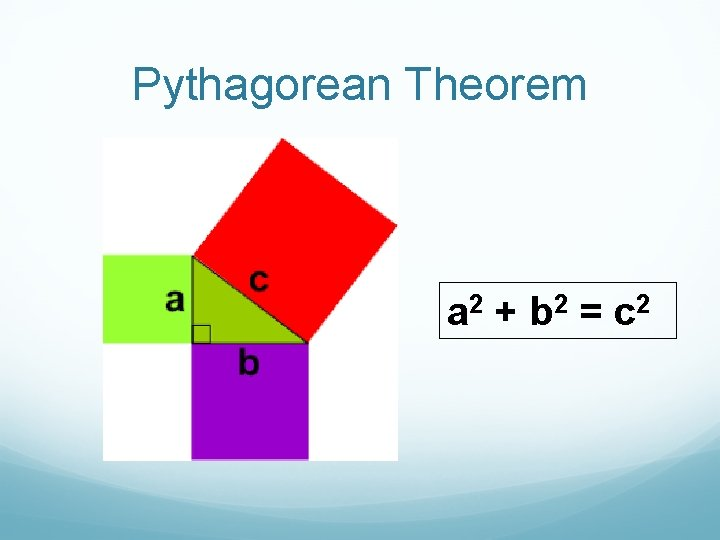Pythagorean Theorem a 2 + b 2 = c 2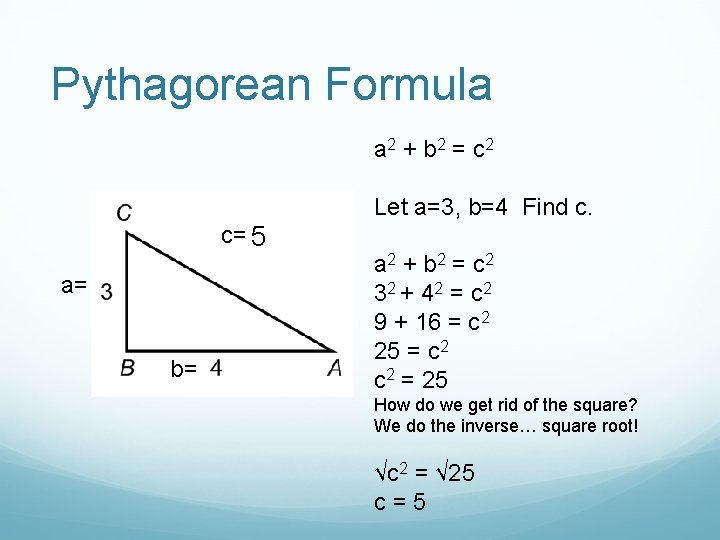Pythagorean Formula a 2 + b 2 = c 2 Let a=3, b=4 Find c. c= 5 a= b= a 2 + b 2 = c 2 32 + 4 2 = c 2 9 + 16 = c 2 25 = c 2 = 25 How do we get rid of the square? We do the inverse… square root! √c 2 = √ 25 c=5Pythagorean Theorem a 2 + b 2 = c 2 a 2 = c 2 - b 2 = c 2 - a 2Word Problems – Ex 1 A boat is 84 m due south of a dock. Another boat is 35 m due east of the dock. How far apart are the boats?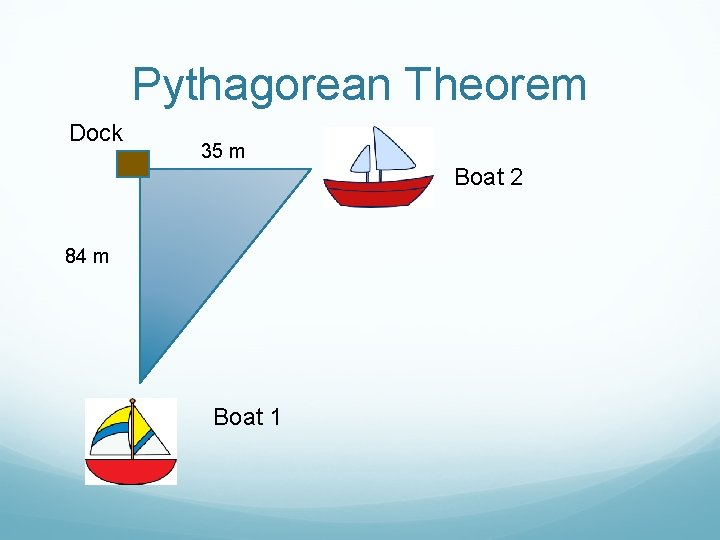Pythagorean Theorem Dock 35 m Boat 2 84 m Boat 1Pythagorean Theorem 35 m a 2 + b 2 = c 2 Let a=84, b=35 c=? 84 m 842 + 352 = c 2 7056 + 1225 = c 2 8281 = c 2 = 8281 √c 2 = √ 8281 c = 91 Therefore the distance between the boats is 91 meters.Example 2 There is a 15 meter ladder that leans against a house. It is 9 meters from the base of the wall. h=? 15 m How high does the ladder reach? 9 m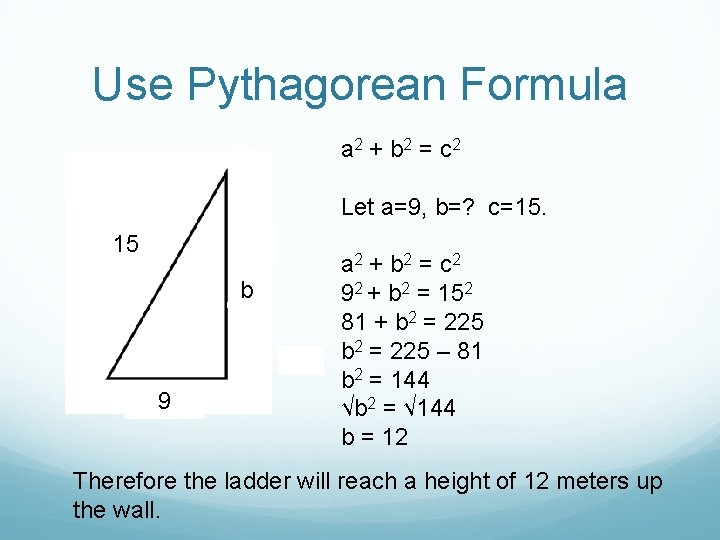Use Pythagorean Formula a 2 + b 2 = c 2 Let a=9, b=? c=15. 15 b 9 a 2 + b 2 = c 2 92 + b 2 = 152 81 + b 2 = 225 – 81 b 2 = 144 √b 2 = √ 144 b = 12 Therefore the ladder will reach a height of 12 meters up the wall.Pythagorean Triples A set of 3 whole numbers that satisfy the Pythagorean Theorem c= 5 3 -4 -5 Triangle a= b= 32 + 42 = 9 + 16 = 25 52 = 25 Left side = Right side!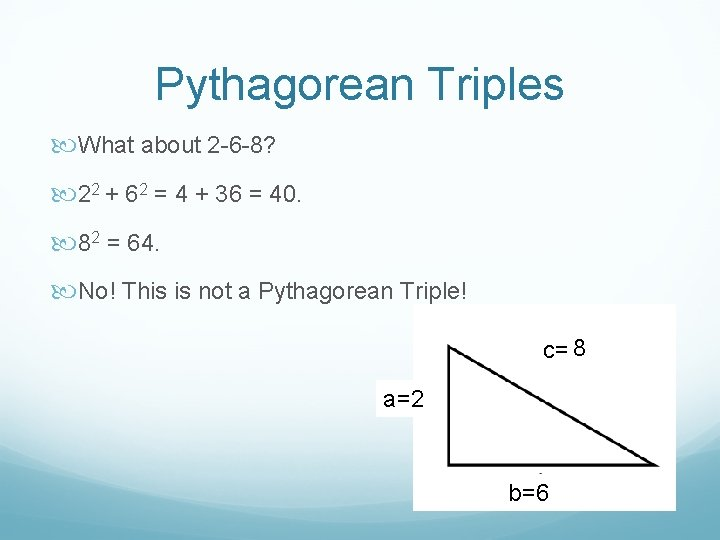Pythagorean Triples What about 2 -6 -8? 22 + 62 = 4 + 36 = 40. 82 = 64. No! This is not a Pythagorean Triple! c= 8 a=2 b=6